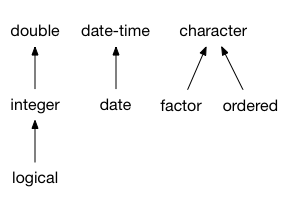vec_ptype2() finds the common type for a pair of vectors, or dies trying. It forms the foundation of the vctrs type system, along with vec_cast(). This powers type coercion but should not usually be called directly; instead call vec_ptype_common().

# S3 method for logical
vec_ptype2(x, y, ...)

# S3 method for integer
vec_ptype2(x, y, ...)

# S3 method for double
vec_ptype2(x, y, ...)

# S3 method for complex
vec_ptype2(x, y, ...)

# S3 method for character
vec_ptype2(x, y, ...)

# S3 method for raw
vec_ptype2(x, y, ...)

# S3 method for list
vec_ptype2(x, y, ...)

vec_ptype2(x, y, ..., x_arg = "x", y_arg = "y")

vec_default_ptype2(x, y, ..., x_arg = "x", y_arg = "y")

## Arguments

x, y Vector types. These dots are for future extensions and must be empty. Argument names for x and y. These are used in error messages to inform the user about the locations of incompatible types (see stop_incompatible_type()).

## Coercion rules

vctrs thinks of the vector types as forming a partially ordered set, or poset. Then finding the common type from a set of types is a matter of finding the least-upper-bound; if the least-upper-bound does not exist, there is no common type. This is the case for many pairs of 1d vectors.

The poset of the most important base vectors is shown below: (where datetime stands for POSIXt, and date for Date)## S3 dispatch

vec_ptype2() dispatches on both arguments. This is implemented by having methods of vec_ptype2(), e.g. vec_ptype2.integer() also be S3 generics, which call e.g. vec_ptype2.integer.double(). vec_ptype2.x.y() must return the same value as vec_ptype2.y.x(); this is currently not enforced, but should be tested.

Whenever you implement a vec_ptype2.new_class() generic/method, make sure to always provide vec_ptype2.new_class.default(). It should normally call vec_default_ptype2().

See vignette("s3-vector") for full details.